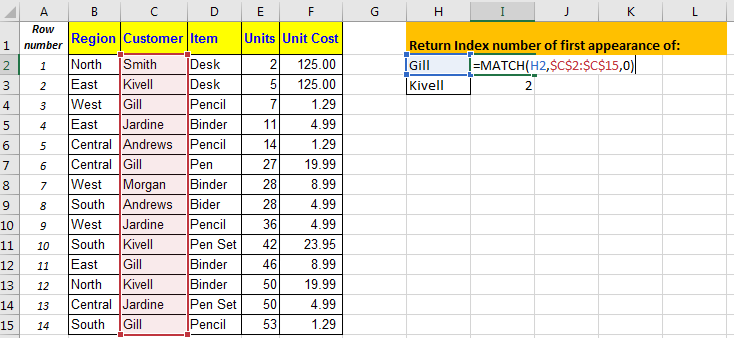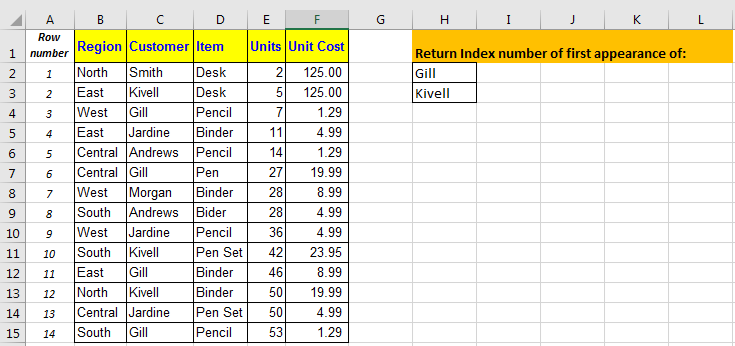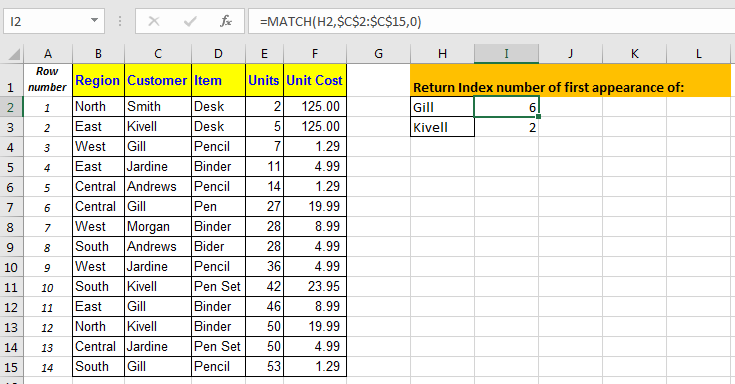# How to Use MATCH Function in Excel

The MATCH function in excel just returns the index of first appearance of a value in an array (single dimension). This function is mostly associated with INDEX function, often known as INDEX-MATCH function.

Let’s understand the aspects of MATCH function.Syntax of MATCH Function:

=MATCH(lookup value, lookup array, match type)

lookup value: The value you are looking for in the array.
lookup array: The arrays in which you are looking for a value
Match Type: The match type. 0 for an exact match, 1 for less than and -1 for greater than.

Example.In the above image, we have a table. In cell H1, we have our task returning Index number of first appearance of Gill and Kivell.

In cell I2, write this formula and drag it down to I3.

=MATCH(H2,\$C\$2:\$C\$15,0)

We have our index numbers.Now you can use this index number with INDEX function of excel to retrieve data from tables.

If you don’t know how to use INDEX MATCH function to retrieve data, below article will be helpful for you.

Retrieving Matching Values from not adjacent List

Do you know how to use MATCH formula with VLOOKUP to automate reports? In Next article we will learn use of VLOOKUP MATCH formula. Till then, Keep Practicing - Keep Excelling.